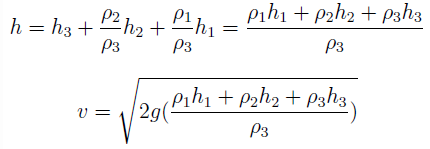Courses

# Test: Hydrostatic Force on Plane Area - 2

## 10 Questions MCQ Test Fluid Mechanics | Test: Hydrostatic Force on Plane Area - 2

Description
This mock test of Test: Hydrostatic Force on Plane Area - 2 for Civil Engineering (CE) helps you for every Civil Engineering (CE) entrance exam. This contains 10 Multiple Choice Questions for Civil Engineering (CE) Test: Hydrostatic Force on Plane Area - 2 (mcq) to study with solutions a complete question bank. The solved questions answers in this Test: Hydrostatic Force on Plane Area - 2 quiz give you a good mix of easy questions and tough questions. Civil Engineering (CE) students definitely take this Test: Hydrostatic Force on Plane Area - 2 exercise for a better result in the exam. You can find other Test: Hydrostatic Force on Plane Area - 2 extra questions, long questions & short questions for Civil Engineering (CE) on EduRev as well by searching above.
QUESTION: 1

### The greatest and the least depth of a circular plate of 4 m diameter from the free surface of water are 3m and 1 m respectively as shown. What will be the total pressure in (kN) on the plate?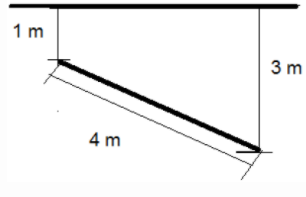Solution:

Explanation: Total liquid pressure on the lamina = F = γ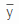A, where γ = specific weight of the liquid,= depth of centroid of the lamina from the free surface, A= area of the centroid. Now, γ = 9.81 * 103 N / m3= 1 + 3 – 1 / 2 – 2m, A = π ⁄ 4 * 42 = 4π m2. Hence, F = 246.55 kN.

QUESTION: 2

### The greatest and the least depth of a circular plate of 4 m diameter from the free surface of water are 3m and 1 m respectively as shown. What will be the depth (in m) of it’s centre of pressure?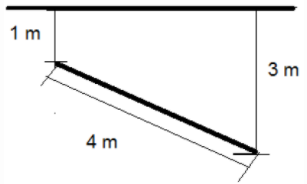Solution:

Explanation: The depth of the centroid y and the centre of pressure yCP are related by: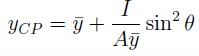where I= the moment of inertia and A = area and θ = the angle of inclination of the lamina to the horizontal. Now,
y = 1 + 3 – 1 / 2 = 2, I = π ⁄ 64 * 42 = 4π, A = π ⁄ 4 * 42 = 4π, sin θ = 1 ⁄ 2 Thus, yCP = 2.125m.

QUESTION: 3

### The highest and lowest vertices of a diagonal of a square lamina (each side equal to 4m) are 1 m and 3 m respectively as shown. What will be the water force (in kN) on the lamina?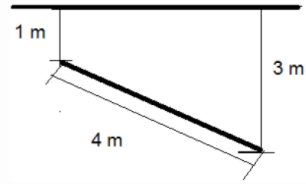Solution:

Explanation: Total liquid pressure on the lamina = F = γA, where γ = specific weight of the liquid,= depth of centroid of the lamina from the free surface, A= area of the centroid. Now, γ = 9.81 * 103 N / m3= 1 + 3 – 1 / 2 = 2m, each side of the lamina =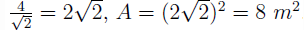Hence, F = 156:96 kN.

QUESTION: 4

The highest and lowest vertices of a diagonal of a square lamina (each side equal to 4m) are 1 m and 3 m respectively as shown. What will be the depth (in m) of it’s centre of pressure?Solution:

Explanation: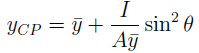where I = the moment of inertia and A= area and θ = the angle of inclination of the lamina to the horizontal. Now,= 1 + 3 – 1 / 2 = 2m, each side of the lamina = =8; sin θ = 3-1/4 = 1 ⁄ 2. Thus, yCP = 2.08m.

QUESTION: 5

A square lamina (each side equal to 2m) is submerged vertically in water such that the upper edge of the lamina is at a depth of 0.5 m from the water surface. If the pressure on the surface is 12 bar, what will be the total water pressure (in kN) on the lamina?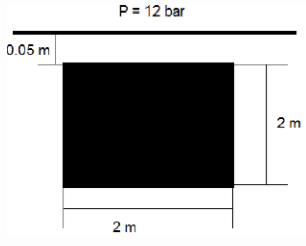Solution:

Explanation: Total liquid pressure on the lamina = F = γA, where γ = specific weight of the liquid,= depth of centroid of the lamina from the free surface, A= area of the centroid. Now, γ = 9.81 * 103 N / m3;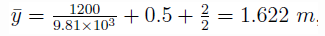A = 2 * 2 = 4 m2. Hence, F = 63.65 kN.

QUESTION: 6

A container is lled with two liquids of densities ρ1 and ρ2 up to heights h1 and h2respectively. What will be the hydrostatic force (in kN) per unit width of the lower face AB?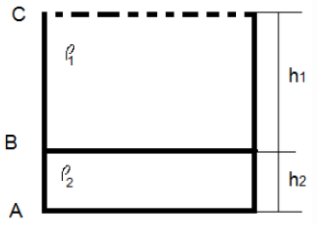Solution:

Explanation: Total liquid pressure on the lamina = F = γA, where γ = specific weight of the liquid,= depth of centroid of the lamina from the free surface, A= area of the centroid. Now,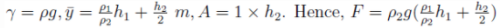QUESTION: 7

A container is lled with two liquids of densities ρ and 2ρ up to heights h and eh respectively. What will be the ratio of the total pressure on the lower face AB and on the upper face BC?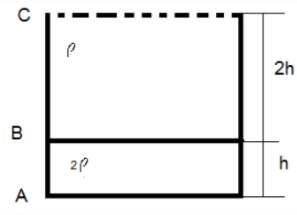Solution:

Explanation: Total liquid pressure on the lamina = F = γA, where γ = specific weight of the liquid,= depth of centroid of the lamina from the free surface, A= area of the centroid.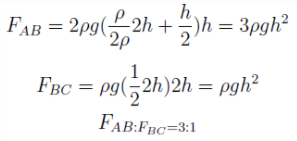QUESTION: 8

A container is lled with two liquids of densities ρ and 2ρ up to heights h and eh respectively. What will be the ratio of the depths of the centres of pressure of the upper face BC and the lower face AB?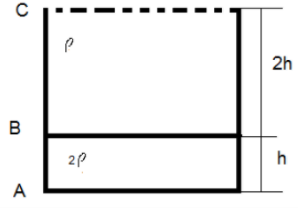Solution:

Explanation: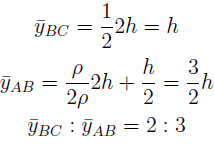QUESTION: 9

A gate of length 5 m is hinged at A as shown to support a water column of height 2.5 m. What should be the minimum mass per unit width of the gate to keep it closed?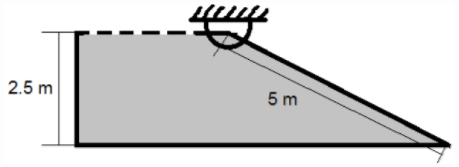Solution:

Explanation: To keep the gate closed, moment due to weight of the gate should be balanced by the moment due to the hydrostatic force.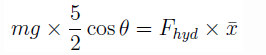where m = mass of the plate, θ = angle of inclination to the horizontal, Fhyd = hydrostatic force on
the plate,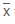= distance of the point of action of Fhyd from the hinge point = 23 * 5 = 103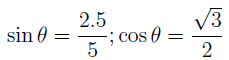Fhyd = γA, where γ = specific weight of the liquid = 9.81 * 103= depth of the centre of pressure from the free surface = 2.5/2 = 1.25 and A = 5 * 1. Substituting all the values in the equation, we get m = 9622.5g.

QUESTION: 10

A large tank is lled with three liquids of densities ρ1, ρ2 and ρ3 up to heights of h1, h2 and h3 respectively. What will be the expression for the instantaneous velocity of discharge through a small opening at the base of the tank? (assume that the diameter of the opening is negligible compared to the height of the liquid column)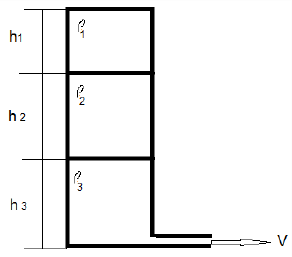Solution:

Explanation: Instantaneous velocity of discharge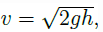where h= height of the liquid column.